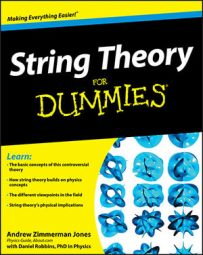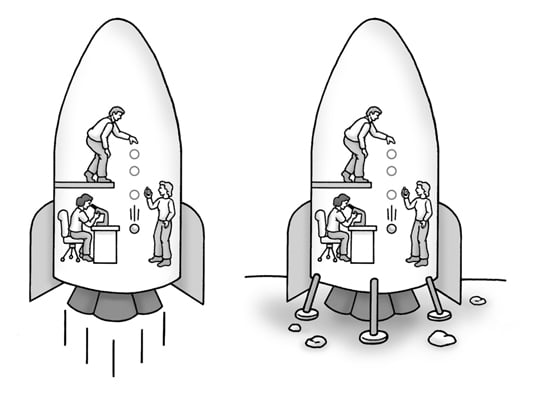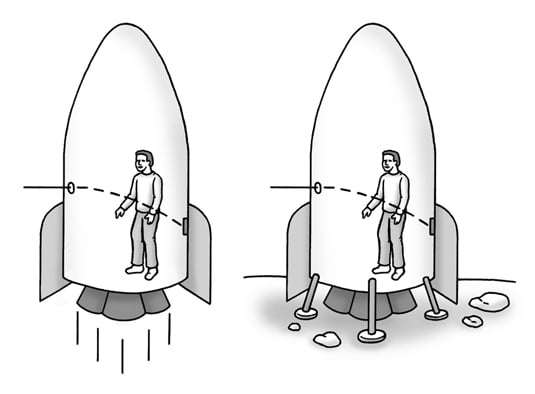##### String Theory For DummiesGeneral relativity was Einstein’s theory of gravity, published in 1915, which extended special relativity to take into account non-inertial frames of reference — areas that are accelerating with respect to each other. General relativity takes the form of field equations, describing the curvature of space-time and the distribution of matter throughout space-time. The effects of matter and space-time on each other are what we perceive as gravity.

Einstein immediately realized that his theory of special relativity worked only when an object moved in a straight line at a constant speed. What about when one of the spaceships accelerated or traveled in a curve?

Einstein came to realize the principle of equivalence, and it states that an accelerated system is completely physically equivalent to a system inside a gravitational field.

As Einstein later related the discovery, he was sitting in a chair thinking about the problem when he realized that if someone fell from the roof of a house, he wouldn’t feel his own weight. This suddenly gave him an understanding of the equivalence principle.

As with most of Einstein’s major insights, he introduced the idea as a thought experiment. If a group of scientists were in an accelerating spaceship and performed a series of experiments, they would get exactly the same results as if sitting still on a planet whose gravity provided that same acceleration, as shown in this figure.(Left) Scientists performing experiments in an accelerating spaceship. (Right) The scientists get the same results after landing on a planet.

Einstein’s brilliance was that after he realized an idea applied to reality, he applied it uniformly to every physics situation he could think of.

For example, if a beam of light entered an accelerating spaceship, then the beam would appear to curve slightly, as in the left picture of the following figure. The beam is trying to go straight, but the ship is accelerating, so the path, as viewed inside the ship, would be a curve.Both acceleration and gravity bend a beam of light.

By the principle of equivalence, this meant that gravity should also bend light, as shown in the right picture of the figure above. When Einstein first realized this in 1907, he had no way to calculate the effect, other than to predict that it would probably be very small. Ultimately, though, this exact effect would be the one used to give general relativity its strongest support.